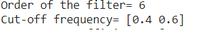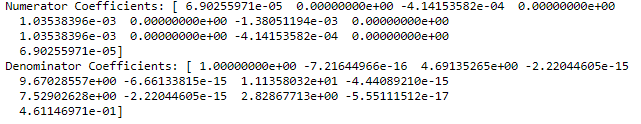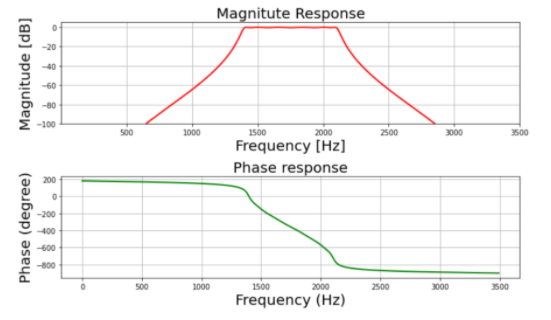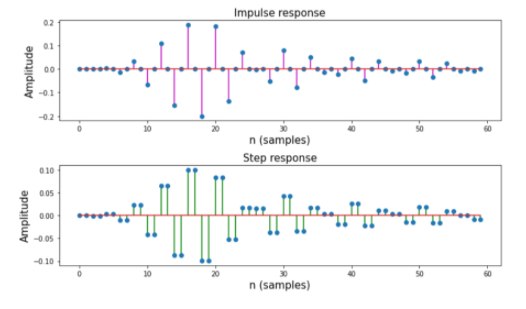Related Articles
Design IIR Bandpass Chebyshev Type-1 Filter using Scipy – Python
• Last Updated : 13 Jan, 2021

IIR stands for Infinite Impulse Response, It is one of the striking features of many linear-time invariant systems that are distinguished by having an impulse response h(t)/h(n) which does not become zero after some point but instead continues infinitely.

## What is IIR Chebyshev Filter?

IIR Chebyshev is a filter that is linear-time invariant filter just like the Butterworth however, it has a steeper roll off compared to the Butterworth Filter. Chebyshev Filter is further classified as Chebyshev Type-I and Chebyshev Type-II according to the parameters such as pass band ripple and stop ripple.

## How is Chebyshev Filter different from Butterworth?

Chebyshev Filter has a steeper roll-off compared to the Butterworth Filter.

## What is Chebyshev Type-I Filter?

Chebyshev Type-I minimizes the absolute difference between the ideal and actual frequency response over the entire passband by incorporating an equal ripple in the passband.

The specifications are as follows:

• Pass band frequency: 1400-2100 Hz
• Stop band frequency: 1050-24500 Hz
• Pass band ripple: 0.4dB
• Stop band attenuation: 50 dB
• Sampling frequency: 7 kHz

We will plot the magnitude, phase, impulse, step response of the filter.

Step-by-step Approach:

Step 1: Importing all the necessary libraries.

## Python3

 `import` `numpy as np``import` `scipy.signal as signal``import` `matplotlib.pyplot as plt`

Step 2:Defining the user defined functions such as mfreqz() and impz().

## Python3

 `def` `mfreqz(b, a, Fs):``   ` `    ``# Compute frequency response of the filter``    ``# using signal.freqz function``    ``wz, hz ``=` `signal.freqz(b, a)`` ` `    ``# Calculate Magnitude from hz in dB``    ``Mag ``=` `20``*``np.log10(``abs``(hz))`` ` `    ``# Calculate phase angle in degree from hz``    ``Phase ``=` `np.unwrap(np.arctan2(np.imag(hz), np.real(hz)))``*``(``180``/``np.pi)``     ` `    ``# Calculate frequency in Hz from wz``    ``Freq ``=` `wz``*``Fs``/``(``2``*``np.pi)``     ` `    ``# Plot filter magnitude and phase responses using subplot.``    ``fig ``=` `plt.figure(figsize``=``(``10``, ``6``))`` ` `    ``# Plot Magnitude response``    ``sub1 ``=` `plt.subplot(``2``, ``1``, ``1``)``    ``sub1.plot(Freq, Mag, ``'r'``, linewidth``=``2``)``    ``sub1.axis([``1``, Fs``/``2``, ``-``100``, ``5``])``    ``sub1.set_title(``'Magnitute Response'``, fontsize``=``20``)``    ``sub1.set_xlabel(``'Frequency [Hz]'``, fontsize``=``20``)``    ``sub1.set_ylabel(``'Magnitude [dB]'``, fontsize``=``20``)``    ``sub1.grid()`` ` `    ``# Plot phase angle``    ``sub2 ``=` `plt.subplot(``2``, ``1``, ``2``)``    ``sub2.plot(Freq, Phase, ``'g'``, linewidth``=``2``)``    ``sub2.set_ylabel(``'Phase (degree)'``, fontsize``=``20``)``    ``sub2.set_xlabel(r``'Frequency (Hz)'``, fontsize``=``20``)``    ``sub2.set_title(r``'Phase response'``, fontsize``=``20``)``    ``sub2.grid()`` ` `    ``plt.subplots_adjust(hspace``=``0.5``)``    ``fig.tight_layout()``    ``plt.show()`` ` `# Define impz(b,a) to calculate impulse response``# and step response of a system``# input: b= an array containing numerator coefficients,``#a= an array containing denominator coefficients``def` `impz(b, a):``   ` `    ``# Define the impulse sequence of length 60``    ``impulse ``=` `np.repeat(``0.``, ``60``)``    ``impulse[``0``] ``=` `1.``    ``x ``=` `np.arange(``0``, ``60``)`` ` `    ``# Compute the impulse response``    ``response ``=` `signal.lfilter(b, a, impulse)`` ` `    ``# Plot filter impulse and step response:``    ``fig ``=` `plt.figure(figsize``=``(``10``, ``6``))``    ``plt.subplot(``211``)``    ``plt.stem(x, response, ``'m'``, use_line_collection``=``True``)``    ``plt.ylabel(``'Amplitude'``, fontsize``=``15``)``    ``plt.xlabel(r``'n (samples)'``, fontsize``=``15``)``    ``plt.title(r``'Impulse response'``, fontsize``=``15``)`` ` `    ``plt.subplot(``212``)``    ``step ``=` `np.cumsum(response) ``     ` `    ``# Compute step response of the system``    ``plt.stem(x, step, ``'g'``, use_line_collection``=``True``)``    ``plt.ylabel(``'Amplitude'``, fontsize``=``15``)``    ``plt.xlabel(r``'n (samples)'``, fontsize``=``15``)``    ``plt.title(r``'Step response'``, fontsize``=``15``)``    ``plt.subplots_adjust(hspace``=``0.5``)`` ` `    ``fig.tight_layout()``    ``plt.show()`

Step 3:Define variables with the given specifications of the filter.

## Python3

 `# Given specification``Fs ``=` `7000`  `# Sampling frequency in Hz``fp ``=` `np.array([``1400``, ``2100``])  ``# Pass band frequency in Hz``fs ``=` `np.array([``1050``, ``2450``])  ``# Stop band frequency in Hz``Ap ``=` `0.4`  `# Pass band ripple in dB``As ``=` `50`  `# stop band attenuation in dB`

Step 4: Computing the cut-off frequency

## Python3

 `# Compute pass band and stop band edge frequencies``wp ``=` `fp``/``(Fs``/``2``)  ``# Normalized passband edge frequencies w.r.t. Nyquist rate``ws ``=` `fs``/``(Fs``/``2``)  ``# Normalized stopband edge frequencies`

Step 5: Compute cut-off frequency & order

## Python3

 `# Compute order of the  Chebyshev type-1 filter using signal.cheb1ord``N, wc ``=` `signal.cheb1ord(wp, ws, Ap, As)`` ` `# Print the order of the filter and cutoff frequencies``print``(``'Order of the filter='``, N)``print``(``'Cut-off frequency='``, wc)`

Output:Step 6: Compute the filter co-efficient

## Python

 `# Design digital Chebyshev type-1 filter``# using signal.cheby1 function``z, p ``=` `signal.cheby1(N, Ap, wc, ``'bandpass'``)`` ` `# Print numerator and denomerator coefficients``# of the filter``print``(``'Numerator Coefficients:'``, z)``print``(``'Denominator Coefficients:'``, p)`

Output:Step 7: Plotting the Magnitude & Phase Response

## Python3

 `# Call mfreqz to plot the magnitude and phase response``mfreqz(z, p, Fs)`

Output:Step 8: Plotting the Impulse and Step Response

## Python3

 `# Call impz function to plot impulse ``# and step response of the filter``impz(z,p)`

Output:Full Code:

## Python3

 `# import required library``import` `numpy as np``import` `scipy.signal as signal``import` `matplotlib.pyplot as plt`` ` ` ` `def` `mfreqz(b, a, Fs):``   ` `    ``# Compute frequency response of the filter``    ``# using signal.freqz function``    ``wz, hz ``=` `signal.freqz(b, a)`` ` `    ``# Calculate Magnitude from hz in dB``    ``Mag ``=` `20``*``np.log10(``abs``(hz))`` ` `    ``# Calculate phase angle in degree from hz``    ``Phase ``=` `np.unwrap(np.arctan2(np.imag(hz), np.real(hz)))``*``(``180``/``np.pi)``     ` `    ``# Calculate frequency in Hz from wz``    ``Freq ``=` `wz``*``Fs``/``(``2``*``np.pi)``     ` `    ``# Plot filter magnitude and phase responses using subplot.``    ``fig ``=` `plt.figure(figsize``=``(``10``, ``6``))`` ` `    ``# Plot Magnitude response``    ``sub1 ``=` `plt.subplot(``2``, ``1``, ``1``)``    ``sub1.plot(Freq, Mag, ``'r'``, linewidth``=``2``)``    ``sub1.axis([``1``, Fs``/``2``, ``-``100``, ``5``])``    ``sub1.set_title(``'Magnitute Response'``, fontsize``=``20``)``    ``sub1.set_xlabel(``'Frequency [Hz]'``, fontsize``=``20``)``    ``sub1.set_ylabel(``'Magnitude [dB]'``, fontsize``=``20``)``    ``sub1.grid()`` ` `    ``# Plot phase angle``    ``sub2 ``=` `plt.subplot(``2``, ``1``, ``2``)``    ``sub2.plot(Freq, Phase, ``'g'``, linewidth``=``2``)``    ``sub2.set_ylabel(``'Phase (degree)'``, fontsize``=``20``)``    ``sub2.set_xlabel(r``'Frequency (Hz)'``, fontsize``=``20``)``    ``sub2.set_title(r``'Phase response'``, fontsize``=``20``)``    ``sub2.grid()`` ` `    ``plt.subplots_adjust(hspace``=``0.5``)``    ``fig.tight_layout()``    ``plt.show()`` ` `# Define impz(b,a) to calculate impulse response ``# and step response of a system``# input: b= an array containing numerator coefficients,``# a= an array containing denominator coefficients``def` `impz(b, a):``   ` `    ``# Define the impulse sequence of length 60``    ``impulse ``=` `np.repeat(``0.``, ``60``)``    ``impulse[``0``] ``=` `1.``    ``x ``=` `np.arange(``0``, ``60``)`` ` `    ``# Compute the impulse response``    ``response ``=` `signal.lfilter(b, a, impulse)`` ` `    ``# Plot filter impulse and step response:``    ``fig ``=` `plt.figure(figsize``=``(``10``, ``6``))``    ``plt.subplot(``211``)``    ``plt.stem(x, response, ``'m'``, use_line_collection``=``True``)``    ``plt.ylabel(``'Amplitude'``, fontsize``=``15``)``    ``plt.xlabel(r``'n (samples)'``, fontsize``=``15``)``    ``plt.title(r``'Impulse response'``, fontsize``=``15``)`` ` `    ``plt.subplot(``212``)``    ``step ``=` `np.cumsum(response)  ``# Compute step response of the system``    ``plt.stem(x, step, ``'g'``, use_line_collection``=``True``)``    ``plt.ylabel(``'Amplitude'``, fontsize``=``15``)``    ``plt.xlabel(r``'n (samples)'``, fontsize``=``15``)``    ``plt.title(r``'Step response'``, fontsize``=``15``)``    ``plt.subplots_adjust(hspace``=``0.5``)`` ` `    ``fig.tight_layout()``    ``plt.show()`` ` ` ` `# Given specification``Fs ``=` `7000`  `# Sampling frequency in Hz``fp ``=` `np.array([``1400``, ``2100``])  ``# Pass band frequency in Hz``fs ``=` `np.array([``1050``, ``2450``])  ``# Stop band frequency in Hz``Ap ``=` `0.4`  `# Pass band ripple in dB``As ``=` `50`  `# stop band attenuation in dB`` ` ` ` `# Compute pass band and stop band edge frequencies``wp ``=` `fp``/``(Fs``/``2``)  ``# Normalized passband edge frequencies w.r.t. Nyquist rate``ws ``=` `fs``/``(Fs``/``2``)  ``# Normalized stopband edge frequencies`` ` `# Compute order of the  Chebyshev type-1``# filter using signal.cheb1ord``N, wc ``=` `signal.cheb1ord(wp, ws, Ap, As)`` ` `# Print the order of the filter and cutoff frequencies``print``(``'Order of the filter='``, N)``print``(``'Cut-off frequency='``, wc)`` ` `# Design digital Chebyshev type-1 filter using ``# signal.cheby1 function``z, p ``=` `signal.cheby1(N, Ap, wc, ``'bandpass'``)`` ` ` ` `# Print numerator and denomerator coefficients of the filter``print``(``'Numerator Coefficients:'``, z)``print``(``'Denominator Coefficients:'``, p)`` ` `# Call mfreqz to plot the magnitude and phase response``mfreqz(z, p, Fs)`` ` `# Call impz function to plot impulse and ``# step response of the filter``impz(z, p)`

Output:Attention geek! Strengthen your foundations with the Python Programming Foundation Course and learn the basics.

To begin with, your interview preparations Enhance your Data Structures concepts with the Python DS Course.

My Personal Notes arrow_drop_up